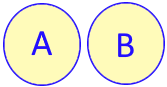# isdisjoint() method for set

All set methods

isdisjoint(set) takes one set ( any iterable ) as argument .

`set` : set to checked
While checking we can use iterable objects like string , list etc Returns : True or False``````A={'x','y','z'}
B={'x','b','c'}
print(A.isdisjoint(B))``````
Output ( 'x' is the common element among two sets A and B )
``False````````A={'x','y','z'}
B={'a','b','c'}
print(A.isdisjoint(B))``````
Output ( There is no common element among two sets A and B )
``True``

## Using stirng

String is an iterable objects
``````A={'x','y','z'}
B='Alex'
print(A.isdisjoint(B))``````
Outut ( here x is the common element )
``False``

## Using list

List is an iterable objects
``````A={'x','y','z'}
B=['a','b','c']
print(A.isdisjoint(B))``````
Output (No common elements )
``True``

Subscribe to our YouTube Channel here

## Subscribe

* indicates required
Subscribe to plus2netplus2net.com